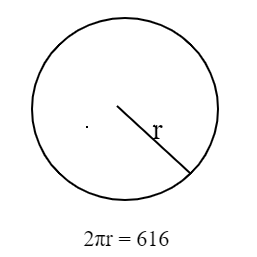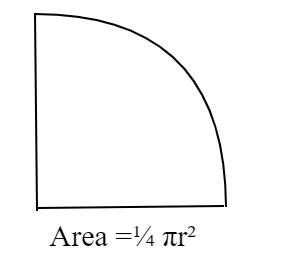QuestionAnswers

# Find the area of a quadrant of a circle whose circumference is 616cm.Hint: In order to solve this problem first find the radius with the help of circumference of the circle. Then find the area by using the formula $\dfrac{{\pi {r^2}}}{4}$. Doing this will solve your problem.The circumference of the circle given is 616cm.
We know that the circumference of the circle is $2\pi r$.
So we can do $2\pi r$= 616cm
$2 \times \dfrac{{22}}{7} \times r = 616{\text{cm}}\,\,\,\,\,\,\,\,\,\,\,\,\,\,\,\,\,\,\,\,\,\,\,\,\,\,\,\left[ {{\text{used}}\,\pi = \dfrac{{22}}{7}} \right] \\ r = \dfrac{{616 \times 7}}{{44}} = 98{\text{cm}} \\$
We know that the area of the quadrant off the circle whose radius is r can be written as:
$\Rightarrow \dfrac{{\pi {r^2}}}{4} \\ \Rightarrow \dfrac{{22 \times {{(98)}^2}}}{{7 \times 4}} = 7546\,{\text{c}}{{\text{m}}^2} \\$
Hence the area of the quadrant is $7546\,{\text{c}}{{\text{m}}^2}$.

Note: Whenever you face such types of problems you need to know that the only parameter we need in a circle is its radius to calculate the area, circumference etc. of any part of it. Proceeding in this way will solve your problem.
View Notes
Area of a Sector of a Circle FormulaArea of a Rhombus FormulaSurface Area of a Prism FormulaHow to Find Square Root of a NumberTo Find the Weight of a Given Body Using Parallelogram Law of VectorsThe Making of a ScientistChanging the Period of a PendulumArea of TrapeziumArea of Hollow CylinderArea of Triangle FormulaImportant Questions for CBSE Class 6 English A Pact with The Sun Chapter 1 - A Tale of Two BirdsImportant Questions for CBSE Class 6 English A Pact with The Sun Chapter 10 - A Strange Wrestling MatchImportant Questions for CBSE Class 6 English A Pact with The Sun Chapter 8 - A Pact with the SunImportant Questions for CBSE Class 6 English Honeysuckle Chapter 8 - A Game of ChanceImportant Questions for CBSE Class 11 Business Studies Chapter 7 - Formation of a CompanyImportant Questions for CBSE Class 7 English Honeycomb Chapter 2 - A Gift Of ChappalsImportant Questions for CBSE Class 6 English Honeysuckle Chapter 5 - A Different Kind of SchoolImportant Questions for CBSE Class 8 Social Science Our Past 3 Chapter 4 - Tribals, Dikus and the Vision of a Golden AgeImportant Questions for CBSE Class 7 English Honeycomb Chapter 10 - The Story of CricketImportant Questions for CBSE Class 6 English A Pact with The SunCBSE Class 10 Hindi A Question Paper 2020Hindi A Class 10 CBSE Question Paper 2009Hindi A Class 10 CBSE Question Paper 2015Hindi A Class 10 CBSE Question Paper 2016Hindi A Class 10 CBSE Question Paper 2012Hindi A Class 10 CBSE Question Paper 2010Hindi A Class 10 CBSE Question Paper 2007Hindi A Class 10 CBSE Question Paper 2013Hindi A Class 10 CBSE Question Paper 2008Hindi A Class 10 CBSE Question Paper 2014RS Aggarwal Class 8 Mathematics Solutions for Chapter-18 Area of a Trapezium and a PolygonRD Sharma Class 7 Solutions Chapter 20 - Mensuration I (Area of Circle) (Ex 20.2) Exercise 20.2RS Aggarwal Solutions Class 8 Chapter-18 Area of a Trapezium and a Polygon (Ex 18B) Exercise 18.2RD Sharma Class 7 Solutions Chapter 20 - Mensuration I (Area of Circle) (Ex 20.1) Exercise 20.1RD Sharma Solutions for Class 9 Maths Chapter 21 - Surface Area and Volume of a SphereRS Aggarwal Solutions Class 8 Chapter-18 Area of a Trapezium and a Polygon (Ex 18A) Exercise 18.1RS Aggarwal Solutions Class 8 Chapter-18 Area of a Trapezium and a Polygon (Ex 18C) Exercise 18.3RD Sharma Solutions for Class 9 Maths Chapter 19 - Surface Area and Volume of A Right Circular CylinderRD Sharma Solutions for Class 9 Maths Chapter 18 - Surface Area and Volume of a Cuboid and CubeRD Sharma Solutions for Class 9 Maths Chapter 20 - Surface Area and Volume of A Right Circular Cone